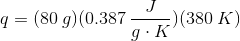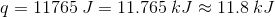# College Chemistry : Calorimetry

## Example Questions

### Example Question #1 : Calorimetry

Which of the following is true of a closed system?

A closed system does not allow for the exchange of matter or energy between the system and its surroundings.

A closed system allows for the exchange of matter between the system and its surroundings, but does not allow the exchange of energy.

A closed system allows for the exchange of energy between the system and its surroundings, but does not allow the exchange of matter.

A closed system allows for the exchange of matter and energy between the system and its surroundings.

A closed system contains no energy or matter and does not allow for the exchange of matter or energy between the system and its surroundings.

A closed system allows for the exchange of energy between the system and its surroundings, but does not allow the exchange of matter.

Explanation:

A closed system allows for the exchange of energy between the system and its surroundings, but does not allow the exchange of matter. This is the definition of a closed system. An open system allows for the exchange of both matter and energy between the system and its surroundings. An isolated system on the other hand does not allow the exchange of either matter or energy between the system and its surroundings.

### Example Question #2 : Calorimetry

When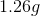of liquid octane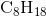undergoes combustion in a bomb calorimeter, the temperature increases from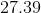degrees Celsius to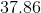degrees Celsius. The heat capacity for the bomb calorimeter is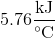. Find the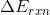for the combustion of octane in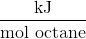.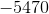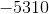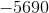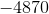Explanation:

Recall the following equation: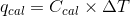Now, since the bomb calorimeter keeps the volume constant, we know the following relationship: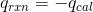Thus, we can then write the following equation for: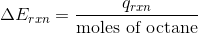Start by finding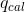: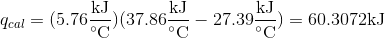From this, we know that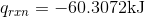Now, find.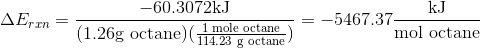Your answer must havesignificant figures.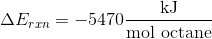### Example Question #3 : Calorimetry

How much heat energy is needed to raise the temperature of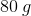of copper from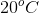to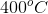? The specific heat capacity of copper is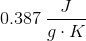.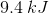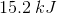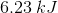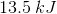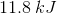Explanation:

In this question, we're given the mass of copper, along with its specific heat capacity, and we're asked to determine the amount of heat energy necessary to increase its temperature by a given amount.

To solve this problem, we'll need to make use of the following equation.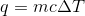Since we know what the values are for the mass and specific heat, we'll need to figure out what the temperature will be. Since the Kelvin and Celsius temperature scales both change by the same amount and only differ at their zero point, we can take the difference of the temperatures in degrees Celsius and use that value (since it will be equivalent to the change in the Kelvin temperature as well).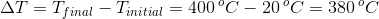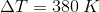Plugging this information into the first expression, we can solve for the amount of heat energy that will bring this mass of copper to the desired temperature.